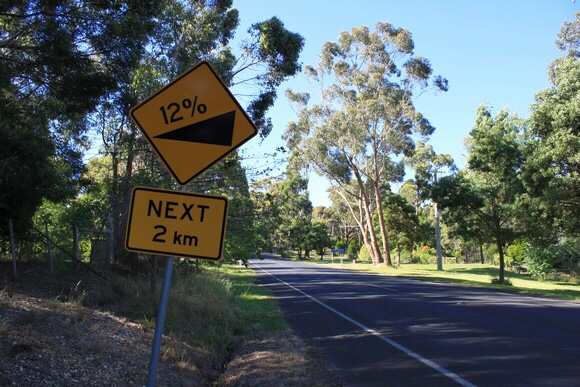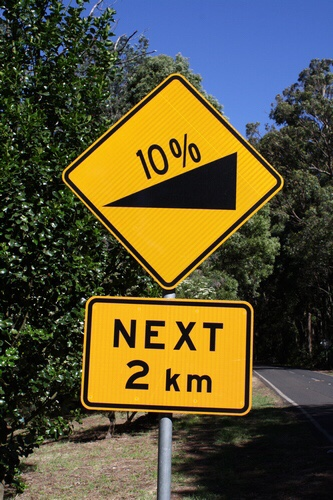# How steep is that hill?

theclimbingcyclist.comIf you’re at all interested in cycling uphill (or even if you’re not) you would have heard people refer to a climb’s gradient (or steepness) as a percentage. A climb might have an average gradient of 3% or 5% or even 10% but just what do these numbers mean? How is gradient calculated? And how challenging are various gradients?

In the first part of this series, we answer these questions and set the scene for more detailed discussions of how gradient applies to cycling.

You might remember from high school maths that gradient is simply defined as rise/run — that is, the distance travelled vertically (b in the diagram below) divided by the distanced travelled horizontally (a in the diagram below). If we want that figure as a percentage then we multiply it by 100.

So let’s say we’ve got a 5km-long climb in which we climb 350 vertical metres. The average gradient is simply:

(350/5000) x 100 = 7%

Right? Almost.

When you climb a hill you aren’t actually riding on the bottom of the right-angled triangle. You are riding on what’s called the hypotenuse, the edge opposite the right angle. That is, the sloping part labelled c in the diagram below.

If we simply divide 350 by 5000, as we did in the example above, we’re dividing the rise (the elevation gain, b) by the hypotenuse of the triangle (c), not the run (a). We need a way to find out the horizontal length (a) based on the information we’ve already got. And to do that we need to employ a basic formula from high school maths.You might remember the Pythagorean Theorem which shows the relationship between the lengths of the sides of a right-angled triangle. Namely:

a² + b² = c²

Remember, c is the length of the hypotenuse and a and b are the lengths of the other two sides. So, if we know two of those lengths (and we do: our hill climbs 350m in 5km) we can find out the third.

Plugging our known values into the Pythagorean Theorem we get:

a² + 350²  = 5000²

a² + 122,500 = 25,000,000

We then subtract 122,500 from both sides:

a² = 25,000,000 – 122,500

a² = 24,877,500

Taking the square root of either side we get:

a = 4988

That is, in a 5km-long climb that rises 350m, we’ve travelled 4988m horizontally (imagine the road is perfectly straight).

So now that we know the horizontal distance travelled (a), as opposed to the hypotenuse (c) — the distance on the road — we can calculate the gradient accurately.

As discussed above, the percentage gradient = (rise/run) x 100. So, in our example:

(350/4988) x 100 = 7.02%You’ll notice that this is extremely close to the figure we calculated before. Given the inherent inaccuracy in elevation measurements — either by a bike-mounted GPS unit, online mapping software or other method — this extra accuracy from calculating the gradient properly is virtually redundant.

Even if we’re talking about a super-steep climb — a rise of 1,500 vertical metres of 10km, say — the simple estimation of (1500/10000 ) x 100 (15% average gradient!) is more than accurate enough. Using the proper method really doesn’t give us any extra accuracy when we consider discrepancies in elevation measurements — an average gradient of 15.2% compared with the 15% we can calculate much faster.

So, when we’re talking about almost every road used for cycling, we can use a simple formula of (rise/hypotenuse) x 100 to estimate the road’s average gradient, even if it’s not technically accurate.

And speaking of not being technically accurate, don’t assume that a climb’s average gradient can tell us everything we need to know about how steep the climb is.

Mt. Hotham, for example, is a 30.8km long climb with an average gradient of 4.2%. From that percentage you might assume Mt. Hotham is a reasonably easy climb. But what the 4.2% doesn’t tell you is that the final third of the climb has several sections that hit 10%. By the same token, an average gradient of 4.2% doesn’t tell you that the middle third of the climb rises at less than that.

So let’s try to put all of this into a bit of context. What does climbing a road with a 5% gradient feel like? And how much easier is it than a gradient of 10%?

Of course, it really depends on how strong you are and what gearing you are running. First-time climbers might find hills with a 5% gradient challenging at first, but after a bit of training it will likely take a much higher gradient to create the same sort of challenge. That said, here’s a rough guide to how various gradients might feel:

• 1-3%: Slightly uphill but not particularly challenging. A bit like riding into the wind.

• 4-6%: A manageable gradient that can cause fatigue over long periods.

• 7-9%: Starting to become uncomfortable for seasoned riders, and very challenging for new climbers.

• 10%-15%: A painful gradient, especially if maintained for any length of time

• 16%+: Very challenging for riders of all abilities. Maintaining this sort of incline for any length of time is very painful.

In the next part in this series, we’ll look at ways of objectively measuring how hard various gradients are and what effect a rider’s weight has on climbing speed.

Thanks to Julian Del Beato, Jeremy Austin, Josh Goodall and Marcus Nyeholt for the help and guidance in putting this article together.